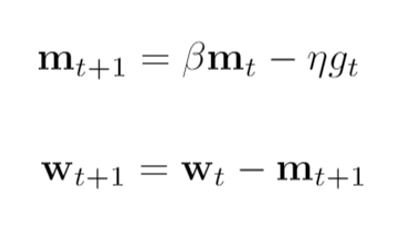# Alternatively train multi task learning model in pytorch - weight updating question

I want to build a multi task learning model on two related datasets with different inputs and targets. The two tasks are sharing lower-level layers but with different header layers, a minimal example:

``````class MultiMLP(nn.Module):
"""
A simple dense network for MTL on hard parameter sharing.
"""
def __init__(self):
super().__init__()
self.hidden = nn.Linear(100, 200)

def forward(self, x):
x = self.hidden(x)
x = F.relu(x)
``````

The dataloader is constructed so that the batches are alternatively generated from two datasets, i.e. batch 0, 2, 4, … from task 0, batch 1, 3, 5, … from task 1. I wanted to train the network in this way: only update weights for `hidden` layer and `out_task0` for batches from task 0, and update only `hidden` and `out_task1` for task 1.

I then alternatively switch `requires_grad` for the corresponding tasks during training as following. But I observed that all weights are updated for every iteration.

``````criterion = MSELoss()
x, y = data, data
controller = i % 2
task0_mode = True if controller == 0 else False
for name, param in model.named_parameters():

outputs = model(x)[controller]
loss = criterion(outputs, y)
loss.backward()
optimizer.step()

for name, p in model.named_parameters():
print(f"Controller: {controller}")
print(name, p)
``````

Did I miss anything in the training procedure? Or the overall setup will not work? Thanks a lot!

2 Likes

The parameters might still get updated even with a zero gradient, if you are using an optimizer with momentum or other running estimates.
Here is a small code example:

``````model = nn.Linear(1, 1, bias=False)

optimizer = torch.optim.SGD(model.parameters(), lr=1., momentum=0.) # same results for w1 and w2
#optimizer = torch.optim.SGD(model.parameters(), lr=1., momentum=0.5) # w2 gets updated
#optimizer = torch.optim.Adam(model.parameters(), lr=1.) # w2 gets updated

w0 = model.weight.clone()

out = model(torch.randn(1, 1))
out.mean().backward()
optimizer.step()
w1 = model.weight.clone()

optimizer.step()
w2 = model.weight.clone()

print(w1 - w0)
print(w2 - w1)
``````

You can comment out/in the different optimizers and will see that `w2` will differ in the second and third use case.

For your use case I think using different optimizers might work best, such that you would only call `optimizer_taskX.step()` using the corresponding optimizer.

4 Likes

Thanks a lot for pointing that out. Indeed, the momentum term would bring the gradients from previous steps.If we zero the gradient at step t, for getting weight at t+1, we still have momentum t.

1 Like

Hi @ptrblck , I’m also having the same questions about how to only train part of network, and thanks for the answer!

One follow-up question is, what is the best-practice of gating in multi-task learning?
The context is that,

• here in OP’s question there are 2 tasks/losses, and gating is desired during training.
• but from OP’s code both tasks are computed for every training example, which is a waste of cpu, because we only want only loss not both.

So should we use an if-else branching inside forward to only activate 1 task? Or what is the best-practice?

I would claim it depends on your use case and especially if you have any knowledge about the task for each sample.
I.e. regardless of the training approach, once the model is trained how would you pass the samples to the model and which predictions do you expect? If each sample also contains information about the task, an if condition inside the `forward` methods could work, since you would be able to use it during training as well as inference. However, if you don’t have the task information during inference, the condition inside `forward` cannot be applied so you wouldn’t be able to deploy the model and might need to stick to calculating the predictions for both tasks (and use more logic to decide which task to pick).

1 Like

Hi @ptrblck , would alternatively detaching each task within the forward method work in this case? Thanks

I’m not sure how detaching is related to to the current issue. Could you explain your idea a bit more, please?

Sorry for being unclear. I mean something like this:

``````class MultiMLP(nn.Module):
"""
A simple dense network for MTL on hard parameter sharing.
"""
def __init__(self):
super().__init__()
self.hidden = nn.Linear(100, 200)

def forward(self, x, detach):
x = self.hidden(x)
x = F.relu(x)
Thanks for the update! If you detach one of the output tensors, you won’t be able to call `backward()` on it and its computation graph won’t be used to calculate the gradients.
In case you are using both tensors to calculate the loss, this could be a valid option. Alternatively, you could also use one of the output tensors to calculate the loss and call `backward` on it.